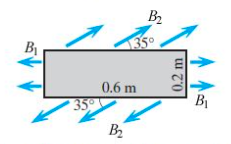# Problem: The figure below shows a measured pattern of magnetic field in space.How much current I passes through the shaded area? (Assume B1 = 7.00x10-5 T and B2 = 8.80x10-4 T.)In what direction?a. out of the pageb. into the pagec. downwardd. upward

###### FREE Expert Solution

In this problem, we're going to use Ampere's law, which is expressed as:

$\overline{){{\mathbf{\mu }}}_{{\mathbf{0}}}{\mathbf{i}}{\mathbf{=}}{\mathbf{B}}{\mathbf{L}}{\mathbf{c}}{\mathbf{o}}{\mathbf{s}}{\mathbf{\theta }}}$, where μ0 is the permeability of free space, is current, B is the magnetic field, L is the length of the segment, and θ is the angle between L and B.

From the figure in the problem, we see that the angle between the magnetic field and length with respect to the upper and lower segments is 35°.

The angle of the magnetic field with respect to the side is 90°.

93% (468 ratings)###### Problem Details

The figure below shows a measured pattern of magnetic field in space.How much current I passes through the shaded area? (Assume B1 = 7.00x10-5 T and B2 = 8.80x10-4 T.)

In what direction?
a. out of the page
b. into the page
c. downward
d. upward